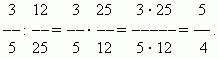# Operations with vulgar fractions

Extension of a fraction. Cancellation of a fraction. Comparison
of fractions. Reducing of fractions to a common denominator.
Addition and subtraction of fractions. Multiplication of fractions.
Division of fractions.

Extension of a fraction. A fraction value isn’t changed, if to multiply its numerator and denominator by the same non-zero number. This transformation of a fraction is called an extension of  a fraction. For instance: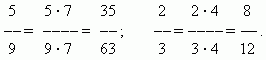Cancellation of a fraction. A fraction value isn’t changed, if to divide its numerator and denominator by the same non-zero number. This transformation of a fraction is called a cancellation of a fraction. For instance: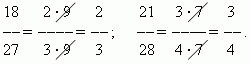Comparison of fractions. From two fractions with the same numerators that one is more, a denominator of which is less: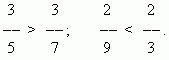From two fractions with the same denominators that one is more, a numerator of which is more: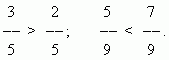To compare two fractions, which have different both numerators and denominators, it is necessary to extend them to reduce to the same denominators.

E x a m p l e . Compare the fractions: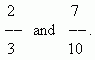S o l u t i o n . Multiply numerator and denominator of the first fraction - by denominator of the second fraction and numerator and denominator of the second fraction - by denominator of the first fraction: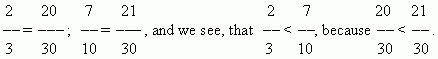The used transformation of fractions is called a reducing of fractions to a common denominator.

Addition and subtraction of fractions. If denominators of fractions are the same, then in order to add the fractions it is necessary to add their numerators; in order to subtract the fractions it is necessary to subtract their numerators (in the same order). The received sum or difference will be a numerator of the result; a denominator is saved the same. If denominators of fractions are different, before these operations it is necessary to reduce fractions to a common denominator. At addition of mixed numbers a sum of integer parts and a sum of fractional parts are found separately. At subtracting mixed numbers we recommend at first to reduce the mixed numbers to improper fractions, then to subtract these fractions and after this to convert the result into a mixed number again (in case of need).

E x a m p l e .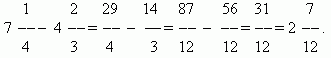Multiplication of fractions. To multiply some number by a fraction means to multiply it by a numerator and to divide a product by a denominator. Hence, we have the general rule for multiplication of fractions : to multiply one fraction by another it is necessary to multiply separately their numerators and denominators and to divide the first product by the second.

E x a m p l e .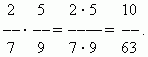Division of fractions. To divide some number by a fraction it is necessary to multiply this number by a reciprocal fraction. This rule follows from the definition of division (see the section "Arithmetical operations" ).

E x a m p l e .